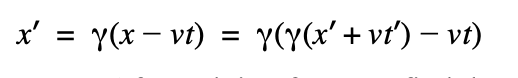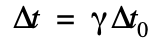top of page
Search

# Relativistic time dilation

ABSTRACT

In this paper, I will demonstrate how the two postulates of Einstein’s special relativity can be used to illustrate the phenomenon of “time dilation,” where time intervals between stationary and moving clocks can differ. I will first describe the scientific context that resulted in the theorization of special relativity, focusing on the absolute speed of light and the problems it creates. I will then use a thought experiment to mathematically derive the formula for time dilation. Finally, I will outline the actual implications of time dilation, what it means, and how it works.

INTRODUCTION

Once Maxwell theorized an absolute and invariant velocity for light, Galilean relativity (and Newtonian mechanics as a whole) was in jeopardy. After all, in classical mechanics, velocity should be a non-invariant quantity, akin to position. To try and reconcile Maxwell’s claim and the Newtonian worldview, scientists postulated the existence of a “luminiferous ether,” which provided the reference frame where light always traveled at a constant velocity. This ether would permeate and drift through the universe, and while light would always have the same velocity with respect to it, the ether drift would allow light to stay non-invariant. The Michelson-Morley experiment was designed to show the existence of the ether, and even find the velocity of it’s drift. To do so, the researchers fired beams of light in various directions, expecting them to travel different velocities as they moved differently through the ether. However, the results showed that light traveled the same velocity regardless of direction. Even after running many trials in many conditions, the speed of light was always constant. Thus the researchers appeared to be left with three conclusions. First, the experiment was flawed. Second, the drift of the ether was somehow always aligned with the movements of the Earth in space. Finally, there was no ether drift, and light really was invariant. While most accepted the first conclusion, Albert Einstein decided to follow the third and develop a new theory of relativity that was aligned with it. Thus, Einstein theorized special relativity, which contained two postulates. The first was identical to Galilean relativity, which was that the laws of physics held true in all inertial reference frames. But the second was that the speed of light was invariant, and would always be “c” regardless of one’s reference frame. By accepting Maxwell’s theory about light, Einstein was able to fashion a new way of analyzing the world around us while still matching empirical observations.

METHODS

Among the many consequences of Einstein’s theory of special relativity is the phenomenon of time dilation. Here, I will show it to be a consequence of the Lorentz transformations. As such, I will derive those transformations first. To do so, consider two separate inertial reference frames, S and S’. In this scenario, frame S is stationary while S’ is moving at constant velocity “v” with respect to S. Now imagine that there is a stationary point P in space, which is at position x in frame S and position x’ in position S’.In classical mechanics, we can convert between the different time and space coordinates of point P with Galilean transformations. If velocity “v” only occurs in the x-direction, they would be as follows:

x' = x - vt

y' = y

t' = t

We see that the distance x’ is simply the sum of x and the distance between the two frames. This distance between frames can be calculated by simply multiplying their relative velocity and the time increment. Since the y-values of the two frames are aligned and there is no y-component of velocity “v,” we see that S and S’ both agree on the y-value of P. Also note that the two reference frames agree on time because in classical mechanics, time is invariant. However, to incorporate special relativity, we must use the Lorentz Transformations.

To find these transformations, consider a similar diagram as above but suppose that at t = t' = 0, which is when the frames overlap, a photon of light is shot forward.How can we convert between reference frames S and S’ while respecting special relativity? Well, we know that distance is the product of rate and time. We also know that the speed of light is “c” for both reference frames. Thus we can state that:

x = ct

x' = ct'

We can also make an assumption that at low velocities, the Lorentz transformations should be approximately equal to the Galilean transformations. As such, we can assume that the Lorentz transformations for x and x’ should be the Galilean ones but with a product that will be denoted as “A.”

x = A(x'+vt')

x' =A(x-vt)

By substituting the definitions of x and x’ in terms of c and t, we find that:

ct = A(ct'+vt')

ct' =A(ct-vt)

This can further be simplified to:

ct = At'(c+v)

ct' =At(c-v)

We wish to find an equation that contains only t or t’ and can do so with the following substitution:Solving for A:Thus we see that A is in fact the Lorentz factor, and that the proper Lorentz transformation for x-coordinates is:As before, we know that velocity “v” only acts in the x-direction, so both S and S’ still agree on the y-position:

y' = y

However, we will now derive the time transformation, as time is no longer invariant. We will do this by substituting the x-coordinate Lorentz transformations into one another:After solving for t, we find that:An analogous process can be used to find t’:Now finally, with these time Lorentz transformations derived, we may also derive time dilation. Suppose again, we have two reference frames S and S’. And yet again, S’ is moving relative to S by the velocity “v.” We would like to find the relationship between a time increment of ∆t for frame S and the equivalent increment ∆t’ for frame S’.

To do this, let us consider an object that is moving with velocity “v” so that it is aligned with the frame S’. In its rest frame S’, the object will experience a certain time interval ∆t’ which should be related to its respective time interval ∆t in its moving frame S. We can model this relationship with the time Lorentz transformation as follows:After simplifying and factoring we see that:However, we know that in the frame S’, the object is at rest. Thus, ∆x’ = 0.Since ∆t’ is the time interval for the object in its rest frame, we may also denote it as ∆t0. Thus we have the formula for time dilation:CONCLUSION

With this equation in derived, we can now analyze its implications. It states that a time interval for a reference frame where an object is in motion is related to a time interval for a reference frame where that same object is stationary by a factor of ɣ.

We also know that ɣ is determined by the relative velocity between these two reference frames, v. Since this velocity must always be less than the speed of light, this means that ɣ must always be greater than or equal to 1. Thus, ∆t must be greater than or equal to ∆t’.This means that the time interval of the moving object’s reference frame is always less than that of an observer’s reference frame. For example, take an object that is travelling relative to a stationary observer at 0.866c.With this parameter, the equation for time dilation states that for every second the travelling object experiences, the stationary observer experiences two. This means that time is no longer absolute, and fluctuates in order to maintain an invariant speed of light. Another noteworthy trait of time dilation is its reciprocity. From each observer’s reference frame, they are the ones that are stationary and the other is the one that is moving. In the example above, from the moving object’s reference frame, it is not moving and the “stationary” observer is moving away from it at 0.866c. By performing calculations identical to the others above, the moving object thus reaches the conclusion that every second for the observer is two seconds for itself.

As such, both observers believe that the other’s clock is running slower, since each believes the other is moving. This symmetry seems counterintuitive, but can be compared to a similar, everyday, phenomenon. If two observers A and B stand a large distance apart, B will appear small to A while A will appear small to B. Due to the effects of perspective, there is no contradiction here even if it may seem like it at first. The symmetry of time dilation is analogous. While it seems contradictory, the effects of special relativity can provide resolutions. However, it is important to remember that special relativity, and thus time dilation, only operates in inertial reference frames. An accelerating object’s reference frame cannot be described by special relativity, and thus its clock can’t be predicted by time dilation.

In conclusion, Einstein’s theory of special relativity has provided us with new tools to describe the world we live in, and has led us to question what we truly know about the universe.

12 views

See All
bottom of page